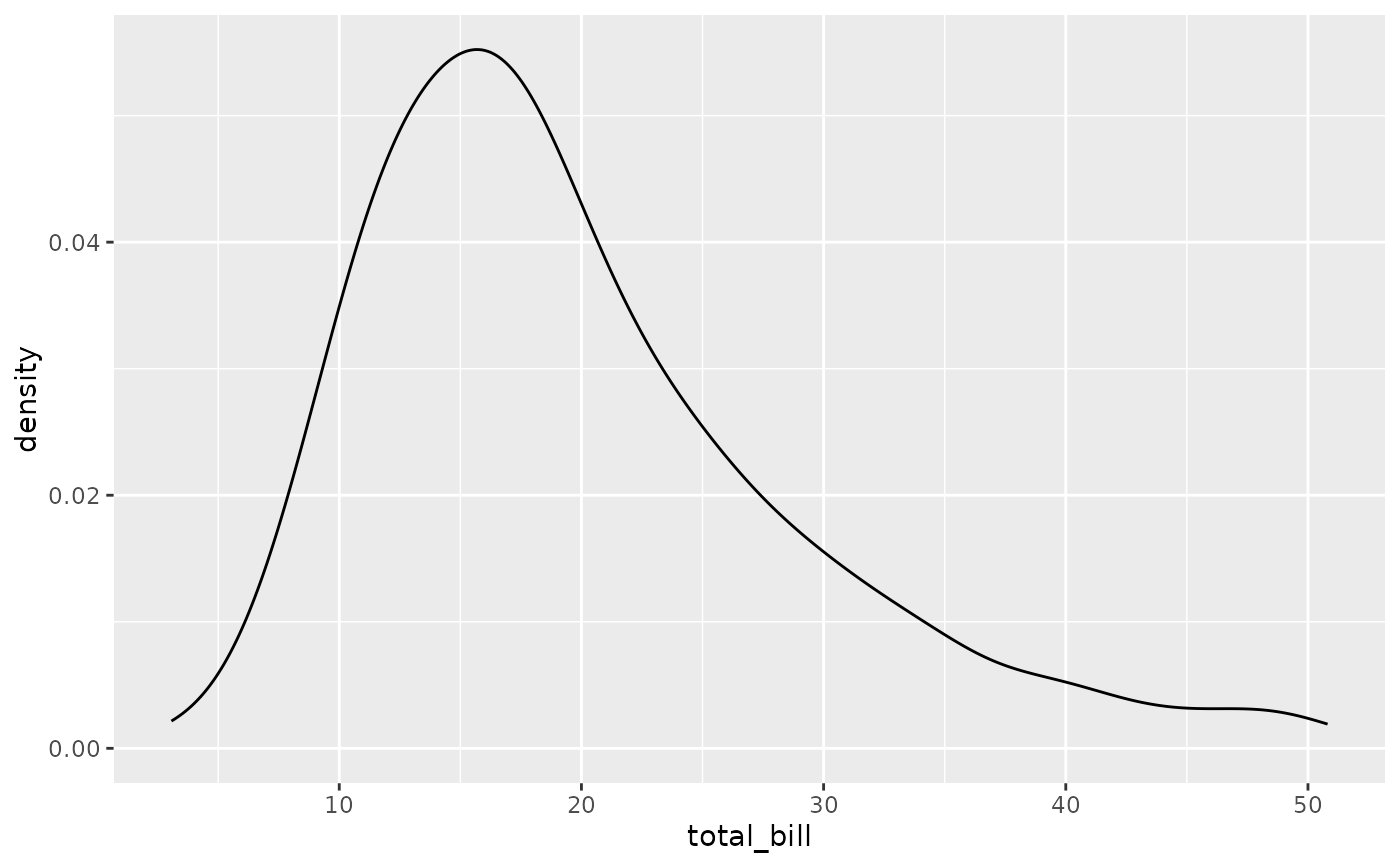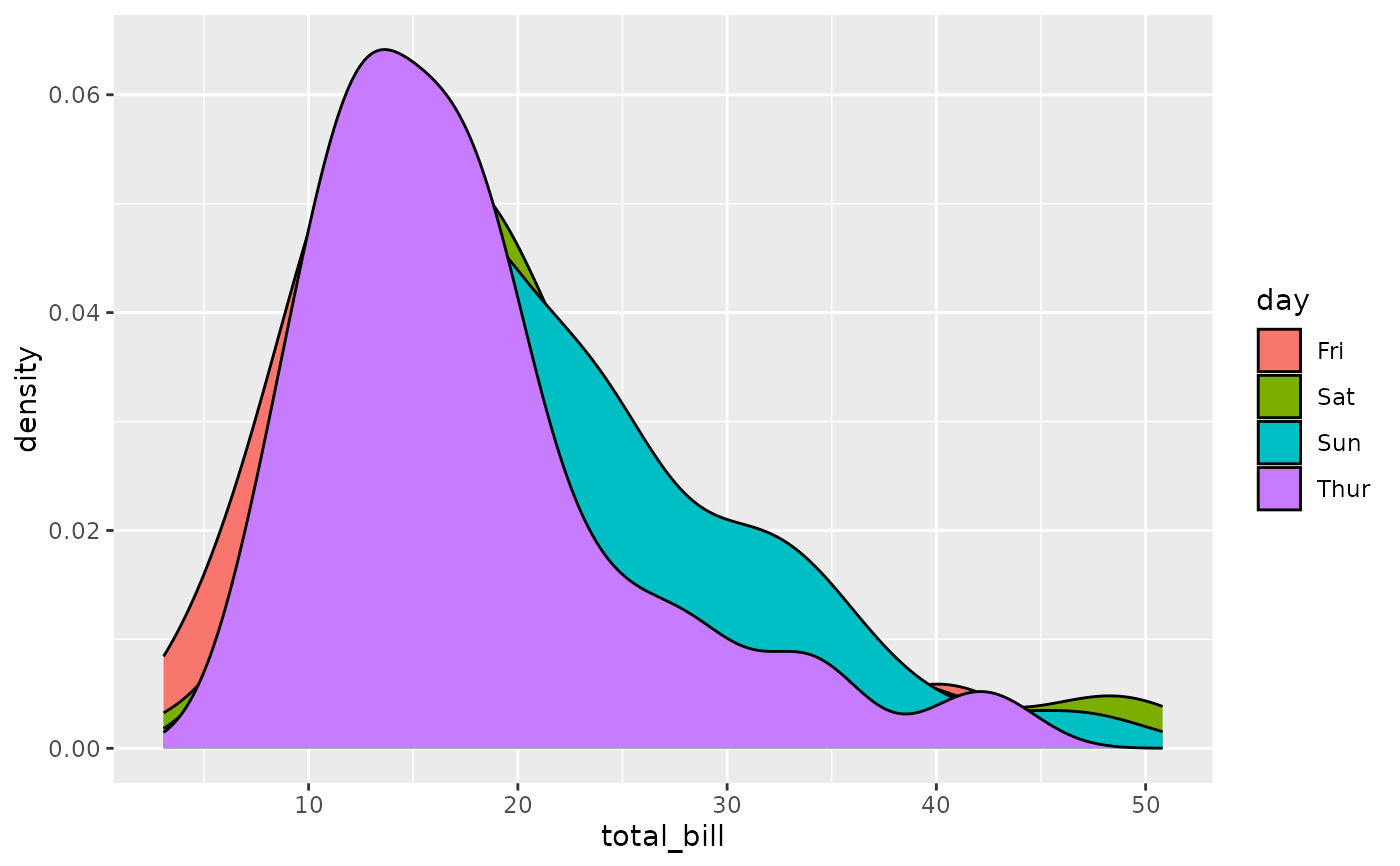Displays a density plot for the diagonal of a ggpairs plot matrix.

ggally_densityDiag(data, mapping, ..., rescale = FALSE)

## Arguments

data data set using aesthetics being used. other arguments sent to stat_density boolean to decide whether or not to rescale the count output

Barret Schloerke

## Examples

# Small function to display plots only if it's interactive
p_ <- GGally::print_if_interactive

data(tips, package = "reshape")
p_(ggally_densityDiag(tips, mapping = ggplot2::aes(x = total_bill)))p_(ggally_densityDiag(tips, mapping = ggplot2::aes(x = total_bill, color = day)))